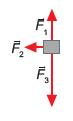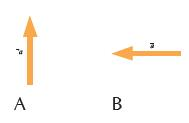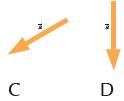Courses

# Olympiad Test : Force, Work And Energy - 1

## 10 Questions MCQ Test Science Olympiad for Class 5 | Olympiad Test : Force, Work And Energy - 1

Description
This mock test of Olympiad Test : Force, Work And Energy - 1 for Class 5 helps you for every Class 5 entrance exam. This contains 10 Multiple Choice Questions for Class 5 Olympiad Test : Force, Work And Energy - 1 (mcq) to study with solutions a complete question bank. The solved questions answers in this Olympiad Test : Force, Work And Energy - 1 quiz give you a good mix of easy questions and tough questions. Class 5 students definitely take this Olympiad Test : Force, Work And Energy - 1 exercise for a better result in the exam. You can find other Olympiad Test : Force, Work And Energy - 1 extra questions, long questions & short questions for Class 5 on EduRev as well by searching above.
QUESTION: 1

Solution:
QUESTION: 2

Solution:
QUESTION: 3

### We do sprinkle powder before playing carom. Why?

Solution:
QUESTION: 4

When the load is nearer the fulcrum than the effort, it is:

Solution:
QUESTION: 5

When the load is further from the fulcrum than the effort, it:

Solution:
QUESTION: 6

You have just kicked a rock, and it is now sliding across the ground, about 2 meters in front of you. Which of these forces act on the ball?

Solution:
QUESTION: 7

Two rubber bands stretched the standard distance cause an object to accelerate at 2 m/s2. Suppose another object with twice the mass is pulled by four rubber bands stretched the standard length. The acceleration of this second object is:

Solution:
QUESTION: 8

Three forces act on an object. In which direction does the object accelerate?Solution:
QUESTION: 9

A simple machine:

Solution:
QUESTION: 10

To press or fasten objects like the book binding press, is an example of:

Solution: